# 7. Classification

`nd` provides a wrapper for `scikit-learn` classifiers that can be directly trained on and applied to `xarray` Datasets.

## 7.1. Training a classifier

First, we need to get some training data. We will do a forest/non-forest classification using some polygon training data, which we can rasterize to match the dataset:

```>>> import nd
>>> from nd.classify import Classifier
>>> from sklearn.ensemble import RandomForestClassifier
>>> path = '../data/C2.nc'
>>> ds = nd.open_dataset(path)
>>> labels = nd.vector.rasterize('../data/labels.shp', ds)['class'].squeeze()
>>> labels.where(labels > 0).plot()
```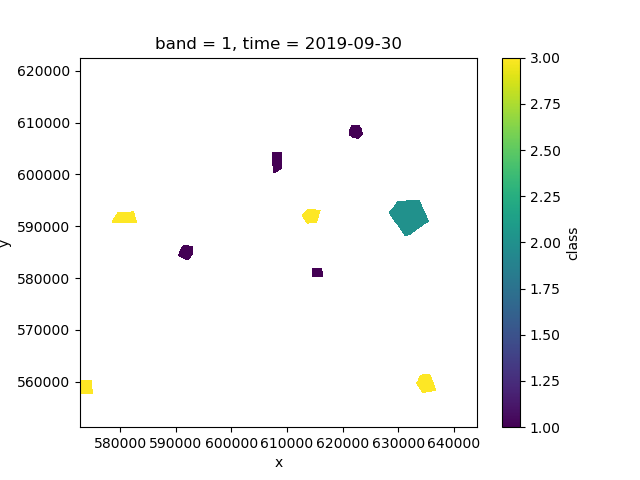If we investigate `labels`, we see that it has an associated legend to match the integer classes:

```>>> labels
<xarray.DataArray 'class' (y: 400, x: 400)>
array([[0, 0, 0, ..., 0, 0, 0],
[0, 0, 0, ..., 0, 0, 0],
[0, 0, 0, ..., 0, 0, 0],
...,
[0, 0, 0, ..., 0, 0, 0],
[0, 0, 0, ..., 0, 0, 0],
[0, 0, 0, ..., 0, 0, 0]])
Coordinates:
band     int64 1
* y        (y) float64 6.225e+05 6.223e+05 6.221e+05 ... 5.515e+05 5.513e+05
* x        (x) float64 5.729e+05 5.73e+05 5.732e+05 ... 6.438e+05 6.44e+05
time     datetime64[ns] 2019-09-30
Attributes:
legend:   [(0, None), (1, 'forest'), (2, 'water'), (3, 'nonforest')]
```
```>>> clf = Classifier(RandomForestClassifier(n_estimators=10))
>>> pred = clf.fit(ds, labels).predict(ds)
>>> pred.isel(time=0).plot()
```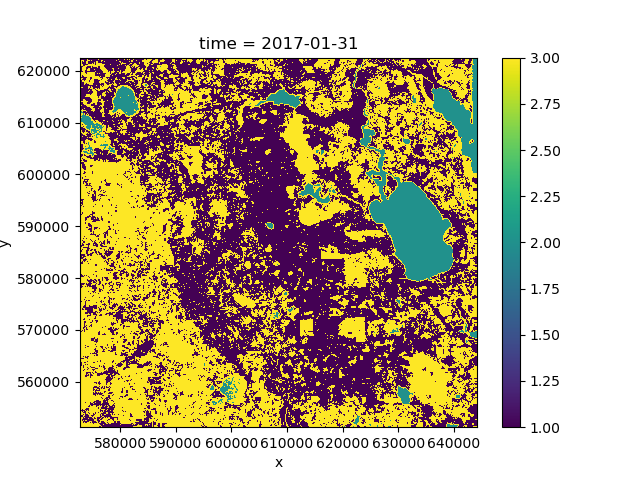If we plot the mean of the predicted class over time we can see that the predictions change because the forest cover changes over the course of the time period:

```>>> pred.mean('time').plot()
```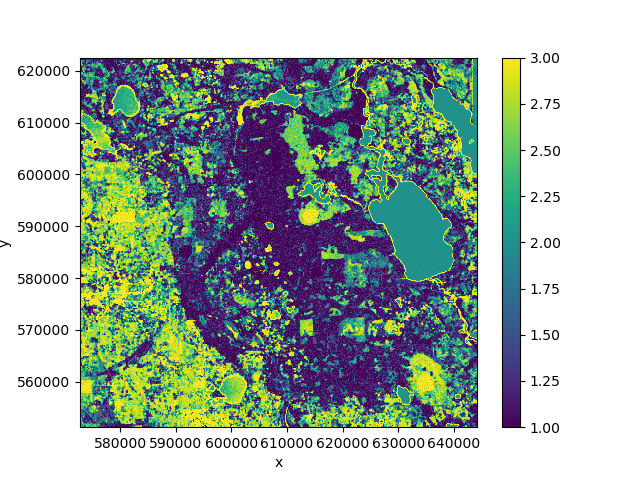## 7.2. Clustering

Clustering can be done using the same `Classifier` object because clustering classes in `scikit-learn` provide the same interface as classifiers. Clustering is an unsupervised approach, so the `labels` argument will be omitted.

In the following example, we are using `nd.classify.class_mean()` to replace every pixel with the mean of its cluster for visualization:

```>>> from sklearn.cluster import MiniBatchKMeans
>>> from nd.classify import class_mean
>>> clf = Classifier(MiniBatchKMeans(n_clusters=3))
>>> pred = clf.fit_predict(ds.isel(time=0))
>>> means = class_mean(ds.isel(time=0), pred)
>>> means.C11.plot()
```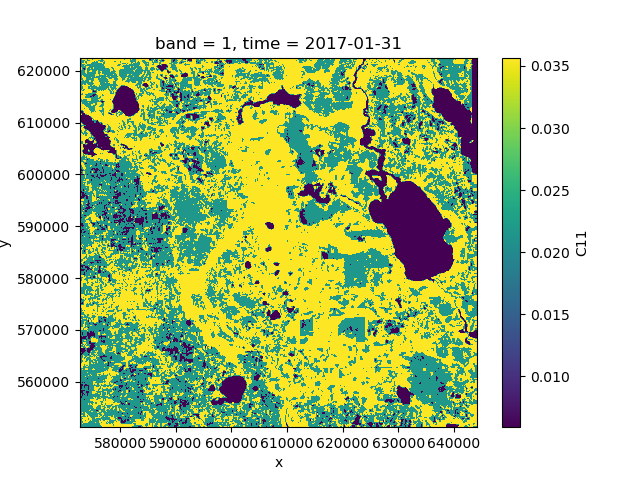It is advisable to use a clustering algorithm that scales well, such as `MiniBatchKMeans`. Alternatively, one can fit the clusterer to a smaller subset of the data by applying a mask.

## 7.3. Feature and data dimensions

Internally, the entire dataset needs to be converted to a two-dimensional array to work with most classification algorithm in `scikit-learn`.

The first dimension (rows) corresponds to independent data points, whereas the second dimension (columns) corresponds to the features (attributes, variables) of that data point.

By default, `nd` will flatten all dataset dimensions into the rows of the array, and convert the data variables into the columns of the array.

However, `nd.classify.Classifier` has an additional keyword argument `feature_dims` that controls which dimensions are considered to be features of the data. Typically, this could be a `band` dimension, which really isn’t a dimensions but a set of features (or variables) of the data. It could also be the `time` dimension, in which case all time steps are treated as additional information about a point, rather than separate points in the feature space.

Example:

```>>> clf = Classifier(RandomForestClassifier(n_estimators=10),
...                  feature_dims=['time'])
>>> pred = clf.fit(ds, labels).predict(ds)
>>> pred.plot()
```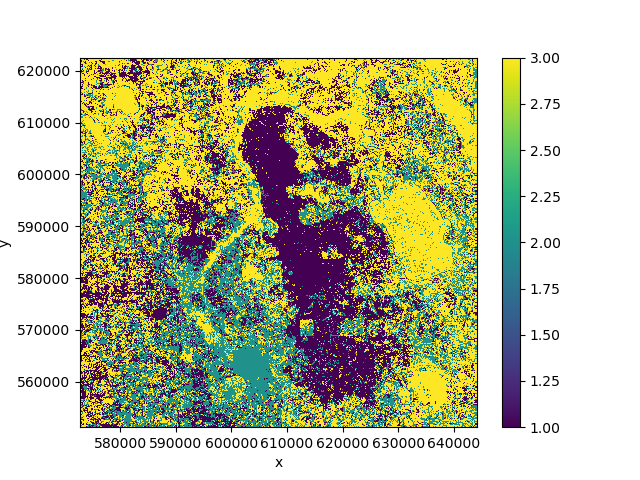Our prediction output no longer has a `time` dimension because it was converted into a feature dimension and used for prediction. In this case the result is not great because the classes change over time and we thus have noisy training data.